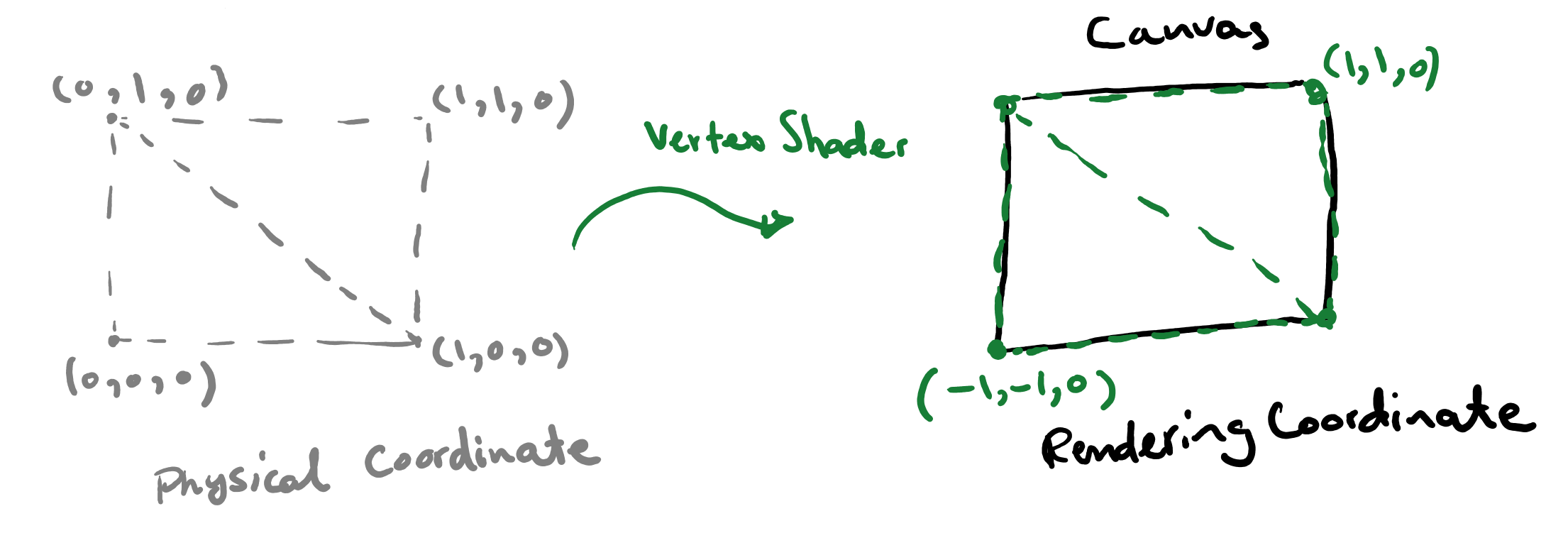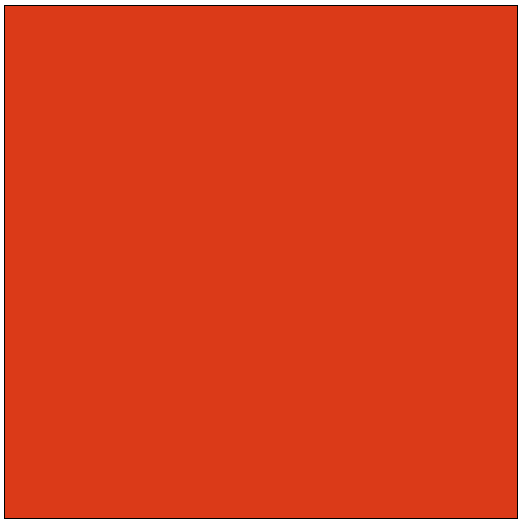Abubu.js
The WebGL Computational Library## Scaled unit rectangle

It will be nice if we can scale the unit rectangle to cover the screen. That way, we can have a custom coordinate system for which the bottom left corner is the origin and the top right corner has coordinate of (1,1). We can achieve this by making minor adjustments to our vertex shader.If the physical system is $$X$$ and $$Y$$, and the rendering coordinates are $$x$$ and $$y$$ our mapping recipe will be

$x = 2X-1,$

and

$y = 2Y-1.$

To apply the above mapping, we modify the vertex shader source from the previous example to be

#version 300 es
precision highp float ; // high percision for float variables
precision highp int ;   // high percision for integer variables

in vec4 position;       // position of vertices as input of the shader

// Main body of the vertex shader
void main() {
/*  an identity map of the position of physical
world coordinates to the rendered coordinate space */
gl_Position = vec4(
position.x*2.-1.,   /* x-coordinate */
position.y*2.-1.,   /* y-coordinate */
position.z,         /* z-coordinate */
1.0);
}


As you can see only the lines where the $$x$$- and $$y$$-coordinates were calculated were modified to include the above-mentioned mapping.

When the above page is opened in a browser, it will produce this:You can also download a copy from the link below by right clicking the link and choosing Save Link As or simply clicking the link to run the program in your browser and then right click in the middle of the page and choose View Page Source to see the full program.

The scaled unit rectangle program